# CSS system Descriptor

The CSS `system` descriptor is used with the `@counter-style` at-rule to define the behavior of the counter style.

The `@counter-style` rule accepts a name, followed by a number of descriptors, which define the counter style. The `system` descriptor is used by the user agent to determine which algorithm will be used to construct a counter's representation based on the counter value.

For example you can make the browser cycle (or loop) through the list of symbols provided, or you could specify that the symbols should be interpreted as digits to an alphabetic numbering system, etc.

## Possible Values

The `system` descriptor accepts the following values.

Below is an explanation of each of these values.

`cyclic`

The `cyclic` system cycles repeatedly through its provided symbols, looping back to the beginning when it reaches the end of the list of symbols.

This system is ideal for simple bullets (using a single counter symbol), but it can also be used to cycle through multiple symbols if required.

Note that if the system is `cyclic`, the `symbols` descriptor must contain at least one symbol.

Here's an example of using a `system` value of `cyclic`.

If your browser supports the `@counter-style` at-rule, the above code could result in a list that looks something like this: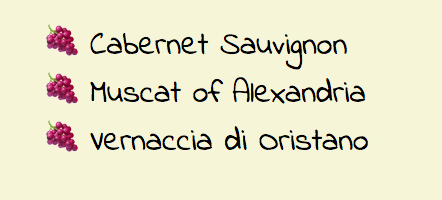An unordered list using the cyclic system (system: cyclic). This example uses unicode emoji character U+1F347 (grapes) for its marker. If the list was 10, 20, or even 50 items long, each item would use a bunch of grapes as its marker. This is because the cyclic system continously loops back to the beginning when it reaches the end of the list of symbols.

Here's another example, except this time using two symbols. The first symbol is the Unicode code point for a red apple. The second is for a green apple. The `system: cyclic` results in the two emojis looping over and over as required to finish the list.

`fixed`

The `fixed` counter system runs through its list of counter symbols once, then falls back to the fallback counter style.

When using the `fixed` counter system, you can add an integer after it to set the first value. If you don't use this, the first value is `1`.

When using the `fixed` counter system, the `symbols` descriptor must contain at least one counter symbol.

Here's an example of using a `system` value of `fixed`.

If your browser supports the `@counter-style` at-rule, the above code could result in a list that looks something like this: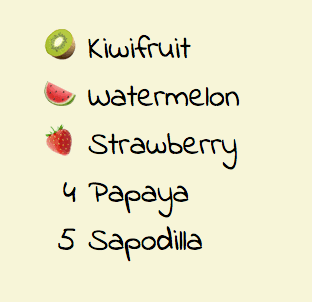An unordered list using the fixed system (system: fixed). This example uses three unicode emoji characters for its markers. These only appear for the first three list items. If there are more than three list items, the marker falls back to the fallback counter style (in this case decimal).
`symbolic`

The `symbolic` counter system cycles repeatedly through its provided symbols, doubling, tripling, etc. the symbols on each successive pass through the list.

For example, if the original symbols were `a`, `b`, and `c` then on the second pass they would instead be `aa`, `bb`, and `cc`, while on the third they would be `aaa`, `bbb`, and `ccc`, etc.

The `symbolic` counter system is ideal for footnotes, but could also be used in other contexts.

If the system is `symbolic`, the `symbols` descriptor must contain at least one counter symbol.

Here's an example of using a `system` value of `symbolic`.

If your browser supports the `@counter-style` at-rule, the above code could result in a list that looks something like this: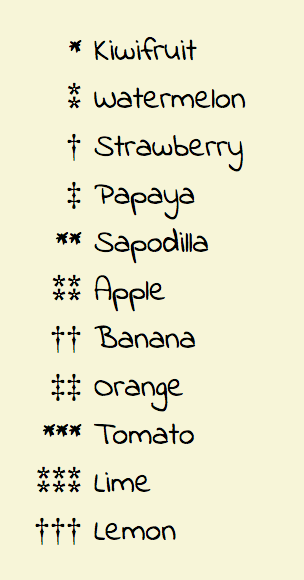An unordered list using the symbolic system (system: symbolic). This example uses four unicode code points for its markers. If the list contains more than four items, the markers appear again but this time adding another one as required. This continues for each iteration until the end of the list.
`alphabetic`

The `alphabetic` counter system interprets the list of counter symbols as digits to an alphabetic numbering system.

For example if `a b c` is provided under `symbols`, the resulting list will go a b c then to aa bb cc then aaa bbb ccc etc.

Alphabetic numbering systems do not contain a digit representing 0. The first counter symbol in the list is interpreted as the digit 1, the second as the digit 2, and so on.

If the system is `alphabetic`, the `symbols` descriptor must contain at least two counter symbols.

Here's an example of using a `system` value of `alphabetic`.

If your browser supports the `@counter-style` at-rule, the above code could result in a list that looks something like this: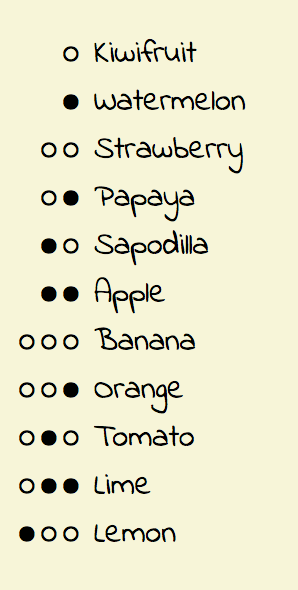An unordered list using the alphabetic system (system: alphabetic). This example uses two unicode characters for its markers. As the list progresses these characters are arranged according to the alphabetic numbering system.
`numeric`

This system interprets the counter symbols as digits in a place-value numbering system. This is similar to the (default) `decimal` system.

The `numeric` system is similar to the `alphabetic` system, with the main difference being that the `numeric` system allows digits that represent 0. In the `numeric` system the the first counter symbol in the list is interpreted as the digit 0, the second as the digit 1, and so on.

If the counter system is `numeric`, the `symbols` descriptor must contain at least two counter symbols.

Here's an example of using a `system` value of `numeric`.

If your browser supports the `@counter-style` at-rule, the above code could result in a list that looks something like this: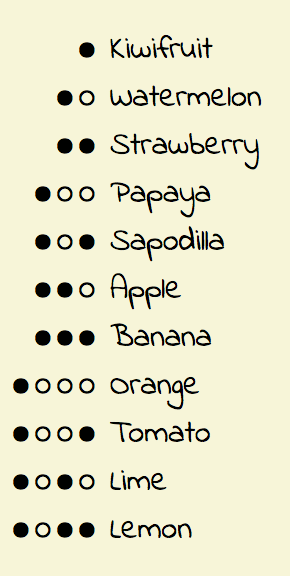An unordered list using the numeric system (system: numeric). This example uses two unicode characters for its markers. As the list progresses these characters are arranged according to the alphabetic numbering system.
`additive`

The `additive` system is used to represent "sign-value" numbering systems (such as Roman numerals) which, rather than reuse digits in different positions to obtain different values, define additional digits for larger values.

If the system is `additive`, the `additive-symbols` descriptor must also be used, and it must contain at least one additive tuple.

An additive tuple is kind of a composite counter symbol, that is made up of two parts, a normal counter symbol and a non-negative integer weight. The additive tuples must be specified in the descending order of their weights.

Here's an example of using a `system` value of `additive`.

If your browser supports the `@counter-style` at-rule, the above code could result in a list that looks something like this: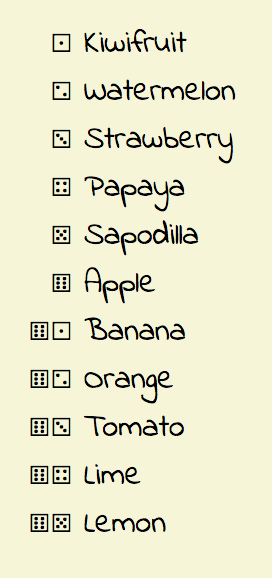An unordered list using the additive system (system: additive). This example uses the unicode code points that represent the die faces 1 to 6 for its markers. As the list progresses these characters are arranged so that the relevant die face is shown. If there are more than six list items, multiple die faces are shown so that they add up to the relevant list item count.
`extends`

The `extends` system allows you to use the algorithm of another counter style, but change other aspects, such as the negative sign or the suffix.

If a `@counter-style` uses the extends system, it must not contain a `symbols` or `additive-symbols` descriptor.

Here's an example of using a `system` value of `extends`.

If your browser supports the `@counter-style` at-rule, the above code could result in a list that looks something like this: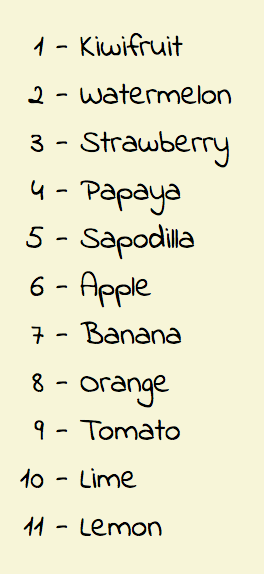An unordered list using the extends system (system: extends decimal) to extend the decimal system. This example replaces the default period (.) with a hyphen (-) when using the decimal system. It also pads the hypen with space on both sides.

## Official Specifications

The `system` descriptor is defined in CSS Counter Styles Level 3 (W3C Candidate Recommendation, 11 June 2015).